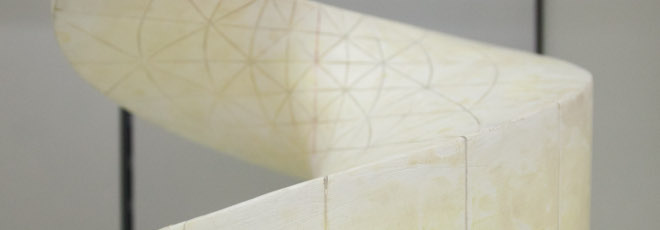## “Shape optimization applied to Eulimnadiae eggs”

Venerdì 12 Giugno 2020, ore 11:00 - Zoom - Alexandre Delyon (Université de Lorraine, Francia)

Abstract

It is reasonable to assume that the form of living organisms observed in nature is optimized to allow them to survive and thrive. The difficulty is essentially to find out what those are optimized for. Shape optimization is a tool that can pretend to answer this question by mathematically modeling biological hypotheses. A shape optimization problem consists in the following: $$\min_{\Omega \in \Omega_{adm}} J(\Omega)$$ where $\Omega_{adm}$ is a class of subset of $\mathbb{R}^N$ and $J : \Omega_{adm} \mapsto \mathbb{R}^N$ is a shape functional.

In this talk we present different approaches to try to explain the shape of the eggs of eulimnadia, a small crustacean of the branchiopod family and similar to a small shrimp. The idea is to turn biological hypothesis into shape optimization problem through a proper modelling. This problem is motivated by the will of biologists to understand the evolutionary history of these animals.

Seminari di equazioni differenziali e applicazioni